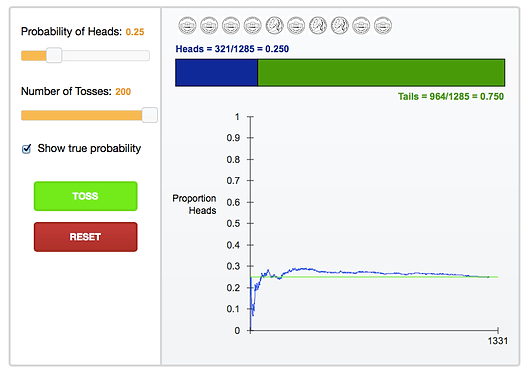top of page

## Chapter 4 - Day 1 - Lesson 4.1

##### Learning Targets
• Interpret probability as a long-run relative frequency.

• Dispel common myths about probability.

• Use simulation to model chance behavior.

##### Activity: Odds or Evens

This lesson took place soon after Halloween, so we brought in candy to give to the winners of today’s game: Odd or Even.  The rules are simple.  Two players where one chooses to be “odds” and the other is “evens”.  Roll two dice.  Find the product of the two dice.  Record whether the product is odd or even.  We modeled a few plays of the game in front of the class, reminding students that product meant multiplication (not sum!).  Students play the game in pairs a  total of 20 times.  The student with the most number of wins out of 20 wins the candy.  We did have one pair of students tie at 10 to 10 so we did a one roll overtime.  Most students will think that the choice of “Odds” or “Evens” doesn’t matter much and that the probability that “Odds” will win is the same as the probability that “Evens” will win.  Simulation shows us otherwise.  As you can see from the results, the probability that “Odds” will win is much lower than for “Evens”.In our discussion at the end of the Activity, we wanted students to notice that there is quite a bit of variability in the winning percentages from one group to another (from 3/20=15% to 10/20=50%), but that when we start to put all of the rolls together as a class (63/200=31.5%), we are getting closer to the actual probability (which is 25%).  This is exactly what the Law of Large Numbers states: that as we simulate more and more trials, our estimate of the probability of “Odds” winning, will approach the true theoretical probability.

We used this Applet to give another visual interpretation of the Law of Large Numbers.  Even though the applet is for tossing coins, it is very easy to change the probability of success to 25% and then see what happens to the estimate of the probability as you add more and more trials.

As for the theoretical probability, it is easy to calculate by writing out all of the possible outcomes in the sample space (which is defined in Lesson 4.2) and simply counting the number of products which are odd. Remember that this method of using the sample space to get a theoretical probability only works if all of the outcomes in the sample space are equally likely.  Also, when students interpret probability, we want them to include the idea of the long run or after many many trials.##### Teaching Tip:

When using Application 4.1: "Will the train arrive on time?" we used question #2 to address the common misconception that people have that due to the “law of averages” there is a better chance that the train will be late on the 6th day because it had been on time the previous 5 days.  We know that if each train is independent from another, that the probability that the train is on time is 0.9 for every train, regardless of the outcome on previous days.  We say “the train has no memory”.  Later in Lesson 4.4, we will discuss more mathematically what it means for two events to be independent.

In question #3, we talked about how to use a random number generator to simulate a situation and estimate a probability.  We reminded students that the Activity of the day (Odd or Even) was also a simulation which helped us to estimate probability.

Question #4 gets students using inferential thinking.  This is yet another preview of significance tests and P-values which will be covered later in the course (Chapter 8).  The big idea is that the outcome observed by the business man (train late 3/20 times) is quite plausible to happen purely by chance if we assume the New Jersey Transit’s 90% claim is true.  We can see this in the dotplot as 30 of the simulations (out of 100) have 3 or more late arrivals.  This proportion 30/100 will later become a P-value for a significance test.

##### Teaching Tip:

It is critical to get students thinking about P-values all through the course.  When we get to Chapter 8, we want students to have a good understanding of a P-value, rather than simply memorizing rules about P-values.  Question #4 of the Application is a really understandable context for starting to develop this thinking.

bottom of page